# AP Statistics : Significance Logic and Establishing Hypotheses

## Example Questions

← Previous 1 3

### Example Question #1 : How To Find P Values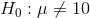and the sample mean is 12.

Select the answer so that both statements indicate a rejection of the null hypothesis at the 95% confidence level.

1) The Z-value for 12 is greater than 1.96

and

2) The p-value for 12 is greater than .05

1) The Z-value for 12 is greater than 1.96

and

2) The p-value for 12 is less than .05

1) The Z-value for 12 is less than 1.96

and

2) The p-value for 12 is less than .05

1) The Z-value for 12 is less than 1.96

and

2) The p-value for 12 is greater than .05

1) The Z-value for 12 is greater than 1.96

and

2) The p-value for 12 is less than .05

Explanation:

In order to reject the null hypothesis, the Z-value for the sample must be greater than (i.e. must lie outside of) the Z-value of the confidence level.

By definition, if the Z-value of the sample is greater than the Z-value of the confidence level, then the p-value of the sample must be less than the p-value for the confidence level.

### Example Question #2 : How To Find P Values

Under the null hypothesis, the distribution of a stock price is normal with meanand standard deviation. The actual stock price now is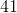. What is the probability that the stock price is this much or greater under the null hypothesis?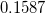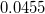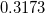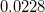Explanation:

This exercise consists of computing the p-value. The null distribution is normal, so we must compute the z-score with the actual data we have and use it to compute the p-value.

We have: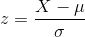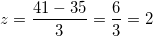Now we calculate the chance that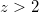, using the context from the problem.

Using a normal table, we get, which is the answer.

### Example Question #3 : How To Find P Values

We are testing the hypothesis that the average gas consumption per day in Billings, Montana is greater than 7 gallons per day; we want 95% confidence.

We sample 30 drivers. The average is 8.4, and the sample standard deviation is 4.29.

Our null hypothesis is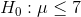1) What is the Z-value for a 1-tailed test at 95%?

2) What is the Z-value for our sample mean of 8.4?

3) What is the p-value for our sample mean of 8.4?

4) Do we reject the null hypothesis?

1) 1.64

2) 1.9

3) .028

4) Yes

1) 1.64

2) 1.95

3) .020

4) Yes

1) 1.64

2) 1.8

3) .036

4) Yes

1) 1.64

2) 1.55

3) .067

4) No

1) 1.64

2) 1.44

3) .083

4) No

1) 1.64

2) 1.8

3) .036

4) Yes

Explanation: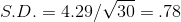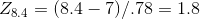From the Z-table: 1.8 corresponds to .9641; p= 1 - .9641 = .036

We reject the null hypothesis since 1.8 > 1.64 (and .036 is less than 95%).

In plain English, we are 95% sure that we will not get a sample mean of 8.4 when the true population mean is 7.

### Example Question #1 : Significance Logic And Establishing Hypotheses

A pretzel company advertises that their pretzels contain less than 1.0g of of sodium per serving. You take a simple random sample of 10 pretzel servings, and calculate that the mean amount of sodium is 1.20 g, with a standard deviation of 0.1 g.

At the 95% confidence level, does your sample suggest that the pretzels actually have higher than 1.0g of sodium per serving?

Yes, because t= 6.32 and p=0.00013694

No, because t=6.32 and p=0.99993153

No, because t=6.32 and p=0.00006847

Yes, because t= 6.32 and p=0.00006847

No, because t=6.22 and p=0.96593153

Yes, because t= 6.32 and p=0.00006847

Explanation:

This is a one- tailed t-test. It is one-tailed because the question asks whether the pretzel's mean is actually higher, so we are only interested in the right hand tail. We will be using the t-distribution because the population standard deviation is not known.

First we write our hypotheses: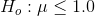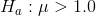Now we need the appropriate formula for a t-test. We will be using standard error because we are working with the standard deviation of a sampling distribution.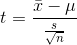Now we fill in the values from our problem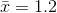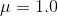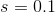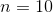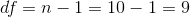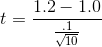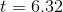Now we must look up the t-critical value, or use technology to find the p-value.

We must find the t-critcal value by finding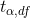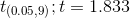Because our test statistic 6.32 is more extreme than our critical value, we reject our null hypothesis and conclude that the pretzels do have a higher mean than 1.0.

If you calculated a p-value using technology, p=0.00006884.

Because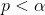, we reject our null hypothesis and conclude that the pretzels do have a higher mean than 1.0 g.

### Example Question #2 : Significance Logic And Establishing Hypotheses

James goes to UCLA, and he believes that the atheletes of UCLA are better runners, than the country average. He did a bit of a research and found that the national average time for a two-mile run for college atheletes ismin with a standard deviation ofminute. He then sampledUCLA atheletes and found that their average two-mile time was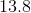minutes.

Is James' data statistically significant? Can we confirm that UCLA atheletes are better than average runners? And if so, to which level of certainty: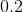,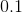,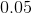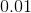Yes, to a certainty ofYes, to a certainty ofNo, the data is not statistically significant

Yes, to a certainty ofYes, to a certainty ofYes, to a certainty ofExplanation:

Using a Z-test (we have population SD, not sample SD) and a population of, we arrive at a P-value of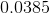, which is lower than, but above.

### Example Question #21 : Ap Statistics

Bob and Alvin suspect that the weight of the average man in Jackson, Mississippi is significantly different than the weight of the average man in Green Bay, Wisconsin. They sample 100 men in Jackson and find that the average weight is 191 lbs, and the sample standard deviation is 30 lbs.

They sample 100 men in Green Bay and find that the average weight is 184, and the sample standard deviation is 25.

Bob says that they should present a 95% 1-tailed test where the alternate hypothesis is: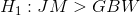and the null hypothesis is: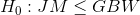Alvin disagrees; he says that a one-tailed test assumes that we already suspected a higher weight in Jackson. He recommends a 95% 2-tailed test where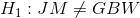and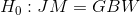.

Show why the 1-tailed test rejects its null hypothesis and the 2-tailed test fails to reject its null hypothesis. Provide the following:

1) The Z-value for a 1-tailed 95% test.

2) The Z-value for a 2-tailed 95% test.

3) The Z-value for the sample difference of 7.

1) 1.645

2) 1.96

3) 1.91

1) 1.645

2) 1.96

3) 1.72

1) 1.645

2) 1.96

3) 1.79

1) 1.645

2) 1.96

3) 1.67

1) 1.645

2) 1.96

3) 1.81

1) 1.645

2) 1.96

3) 1.79

Explanation:

The variance for the sample difference is: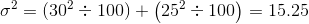The standard deviation for the sample difference is: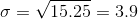The Z-value for a difference of 7 is: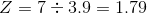The sample difference Z-value is greater than the 1-tailed Z-value (causing us to reject the null hypothesis) and is less than the 2-tailed Z-value (causing us to fail to reject the null hypothesis).

### Example Question #1 : How To Establish An Alternate Hypothesis

A researcher stated thatpercent of Americans in the 2008 presidential election were liberal. The researcher wants to find evidence that the percent of liberal Americans was different for the 2012 presidential election. Which of the hypotheses best describes the question that the researcher is trying to answer?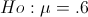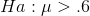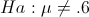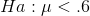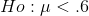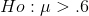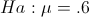Explanation:

The question states that the researcher thinks the percent of liberal Americans in 2012 is different than thepercent in 2008. The hypothesis is 2 directional. Therefore the null hypothesis is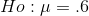and the alternative is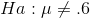.

### Example Question #4 : Significance Logic And Establishing Hypotheses

The department of labor reports that umemployment is down to 5% in the United States.  A politician claims that the data was manipulated to show better numbers. The politician believes our country is worse off that unemployment must be worse than the claim made by the department of labor.  What is the alternative hypothesis for a test for this situation?

Ha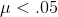Ho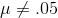Ho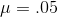Ha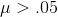HaHaExplanation:

The alternative hypthesis is denoted by Ha. The alternative represents the value that is being tested for and not the original claim.  The original claim for the problem is unemployment is%.  The test claim is that it is greater than%.  This means the alternative must be.

### Example Question #5 : Significance Logic And Establishing Hypotheses

A researcher wants to determine whether there is a significant linear relationship between time spent meditating and time spent studying. What is the appropriate alternative hypothesis for this study?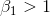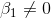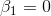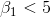Explanation:

This question is about a linear regression between time spent meditating and time spent studying. Therefore, the hypothesis is regarding Beta1, the slope of the line. We are testing a non-directional or bi-directional claim that the relationship is significant. A slope of zero indicates no relationship between the two variables. Therefore, the alternative hypothesis is that the slope is not equal to zero.

### Example Question #6 : Significance Logic And Establishing Hypotheses

A researcher wants to compare 3 different treatments to see if any of the treatments affects study time. The three treatments studied are control group, a group given vitamins, and a group given a placebo.  They found that the average time spent studying with control students was 2 hours, with students given vitamins it was 3 hours, and with placebos students studied 5 hours. Which of the following is the correct alternative hypothesis?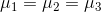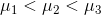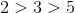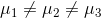Not all of the means are equal

Because we are comparing more than 2 groups, we must use an ANOVA for this problem. For an ANOVA problem, the null hypothesis is that not all of the groups’ means are the same.is not correct because this hypothesis implies that all of the groups are different, which is not actually tested by the basic ANOVA test.